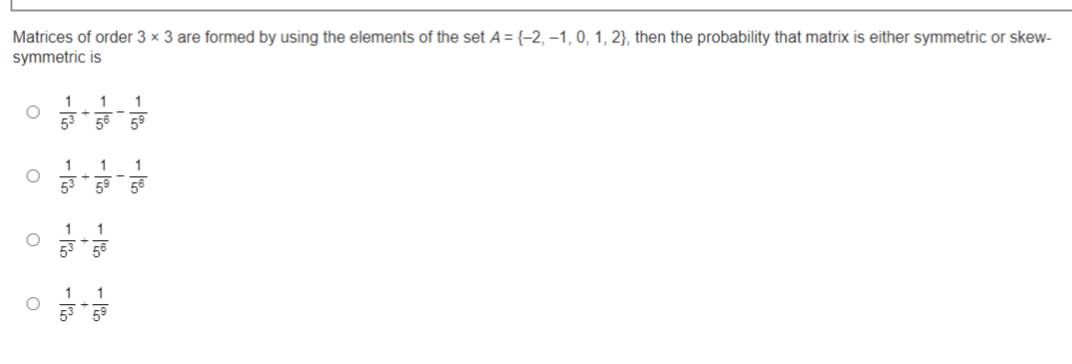# Matrices of order 3*^ , are formed by using the elements of the set A = \{- 2, - 1, 0, 1, 2\} , then the probability that matrix is either symmetric or skew symmetric is

70 viewsby (45.9k points)

Correct option is (A) $\frac1{5^3}+\frac1{5^6}-\frac1{5^9}$

Total no. of matrices of order 3 x 3 formed by using the elements of the set

A = {-2, -1, 0, 1, 2} = 59

($\because$ There are total 9 elements in the matrix and each element have 5 choices)

Total no. of symmetric matrices formed by Set A = {-2, -1, 0, 1, 2} = 56

Total no. of skew symmetric matrices formed by Set A = 53

Total no. of matrices which is both symmetric and skew symmetric formed by using elements of Set A = 1

($\because$ only zero matrix is both symmetric & skew symmetric.)

$\therefore$ Total no. of matrices that is either symmetric or skew symmetric and formed by elements of Set A = Total no. of symmetric matrices  + Total no. of skew symmetric matrices - Total no. of matrices which are both

= 56 + 53 - 1

$\therefore$ required probability = $\frac{5^6+5^3-1}{5^9}$ = $\frac1{5^3}+\frac1{5^6}-\frac1{5^9}$